# 1016 and Level 4

If you’ve never tried a level 4 puzzle before, this is a great one to try. Of its twelve clues, eight have only one factor pair in which both factors are from 1 to 12. You should easily be able to place the factors for those eight clues. Since each factor from 1 to 12 must appear exactly one time in the first column and the top row, the factors from those eight clues will eliminate some of the possible factors of the other four clues. Don’t be afraid to give this puzzle a try!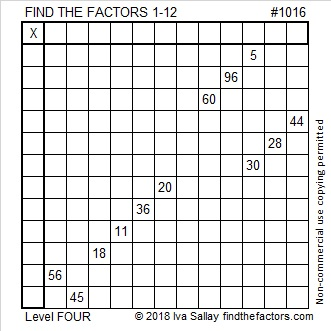Print the puzzles or type the solution in this excel file: 12 factors 1012-1018

Here are some reasons why 1016 is an interesting number:

1016 is the sum of seven consecutive powers of two:
2⁹ + 2⁸ + 2⁷ + 2⁶ + 2⁵ + 2⁴ + 2³ = 1016
I know that’s true because 1016 is 1111111000 in BASE 2

1016 is a palindrome in a couple of bases as well:
It’s 13031 in BASE 5 because 1(5⁴) + 3(5³) + 0(5²) + 3(5¹) + 1(5⁰) = 1016
161 in BASE 29 because 1(29²) + 6(29¹) + 1(29⁰) = 1016

1016 is divisible by 2 because 6 is even.
1016 is divisible by 4 because 16 is divisible by 4. (And also because 6 is divisible by 2 but NOT by 4 and 1 is odd.)
1016 is divisible by 8 because 016 is divisible by 8. (And because 16 is divisible by 8 and 0 is an even number.
1016 is NOT divisible by 16 because 016 is divisible by 16 and 1 is an odd number.

• 1016 is a composite number.
• Prime factorization: 1016 = 2 × 2 × 2 × 127, which can be written 1016 = 2³ × 127
• The exponents in the prime factorization are 3 and 1. Adding one to each and multiplying we get (3 + 1)(1 + 1) = 4 × 2 = 8. Therefore 1016 has exactly 8 factors.
• Factors of 1016: 1, 2, 4, 8, 127, 254, 508, 1016
• Factor pairs: 1016 = 1 × 1016, 2 × 508, 4 × 254, or 8 × 127
• Taking the factor pair with the largest square number factor, we get √1016 = (√4)(√254) = 2√254 ≈ 31.87475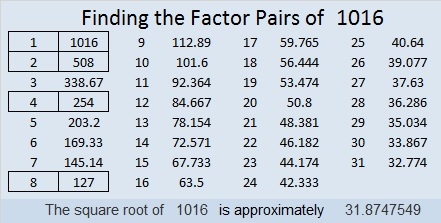# Divisibility Tricks Applied to 693

• 693 is a composite number.
• Prime factorization: 693 = 3 x 3 x 7 x 11, which can be written 693 = (3^2) x 7 x 11
• The exponents in the prime factorization are 2, 1, and 1. Adding one to each and multiplying we get (2 + 1)(1 + 1)(1 + 1) = 3 x 2 x 2 = 12. Therefore 693 has exactly 12 factors.
• Factors of 693: 1, 3, 7, 9, 11, 21, 33, 63, 77, 99, 231, 693
• Factor pairs: 693 = 1 x 693, 3 x 231, 7 x 99, 9 x 77, 11 x 63, or 21 x 33
• Taking the factor pair with the largest square number factor, we get √693 = (√9)(√77) = 3√77 ≈ 26.324893Some quick divisibility tricks applied to the number 693:

1. Every counting number is divisible by 1
2. 693 is not even so it isn’t divisible by 2
3. Every digit of 693 is divisible by 3, so 693 is divisible by 3
4. Since it isn’t divisible by 2, it isn’t divisible by 4
5. 693 doesn’t end in a 5 or 0, so it’s not divisible by 5
6. 693 is divisible by 3 but not by 2 so it isn’t divisible by 6
7. 69 – 2(3) = 63, a multiple of 7 so 693 is divisible by 7
8. Since it isn’t divisible by 2 or 4, it can’t be divisible by 8
9. 6 + 9 + 3 = 18, a multiple of 9 so 693 is divisible by 9
10. Since the last digit of 693 isn’t 0, it is not divisible by 10
11. 69 + 3 = 0, so 693 is divisible by 11

The divisibility tricks that worked on the number 693 are quite easy to see on the outside of this factor cake.Here is today’s factoring puzzle: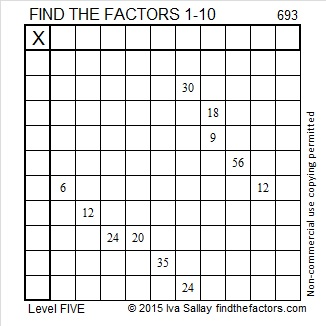Print the puzzles or type the solution on this excel file: 10 Factors 2015-11-23

———————————————————————————

693 is a palindrome in several bases:

• 1010110101 BASE 2; note 1(512) + 0(256) + 1(128) + 0(64) + 1(32) + 1(16) + 0(8) + 1(4) + 0(2) + 1(1) = 693
• 3113 BASE 6; note 3(216) + 1(36) + 1(6) + 3(1) = 693
• 414 BASE 13; note 4(169) + 1(13) + 4(1) = 693
• 313 BASE 15; note 3(225) + 1(15) + 3(1) = 693

———————————————————————————Ricardo tweeted his work for this puzzle, too.

# A Teacher Affects Eternity and Divisibility Tricks Applied to 418

Henry Brooks Adams said, “A teacher affects eternity; he can never tell where his influence stops.” Of course the quote applies to female teachers just as much as it does to male teachers. Affecting eternity is pretty serious business.

It will probably take less than two minutes to read Marek Bennet’s  comic on how the work you do fits into the educational system. Its message is worth pondering for quite a long time.Let me demonstrate some quick prime number divisibility tricks on the number 418:

• It’s even so its divisible by 2.
• 4 + 1 + 8 = 13, which is not a multiple of 3, so 418 is not divisible by 3.
• The last digit isn’t 0 or 5, so 418 is not divisible by 5.
• 41 – 2(8) = 41 – 16 = 25, which is not a multiple of 7, so 418 is not divisible by 7.
• If we add the first and the 3rd digits of 418, we get twelve. Then if we subtract the second digit, one, we get eleven. Since we got a multiple of eleven, that means that our original number, 418, can be evenly divided by eleven.

Here is the factoring information for 418:

• 418 is a composite number.
• Prime factorization: 418 = 2 x 11 x 19
• The exponents in the prime factorization are 1, 1, and 1. Adding one to each and multiplying we get (1 + 1)(1 + 1)(1 + 1) = 2 x 2 x 2 = 8. Therefore 418 has exactly 8 factors.
• Factors of 418: 1, 2, 11, 19, 22, 38, 209, 418
• Factor pairs: 418 = 1 x 418, 2 x 209, 11 x 38, or 19 x 22
• 418 has no square factors that allow its square root to be simplified. √418 ≈ 20.445# 140 and Gr-8 Divisibility Tricks

140 is a composite number. Factor pairs: 140 = 1 x 140, 2 x 70, 4 x 35, 5 x 28, 7 x 20, 10 x 14. Factors of 140: 1, 2, 4, 5, 7, 10, 14, 20, 28, 35, 70, 140. Prime factorization: 140 = 2 x 2 x 5 x 7, which can also be written 140 = 2² x 5 x 7.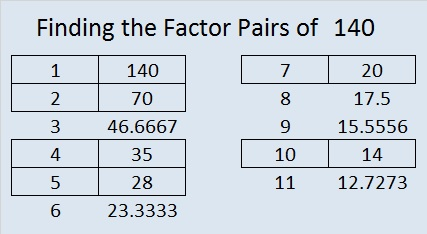140 is never a clue in the FIND THE FACTORS puzzles.

——————————————————————-

In a previous post I discussed how to tell if a whole number can be evenly divided by 4, 25, or both just by looking at the last 2 digits of the number.

Mathematics is full of patterns so you might be wondering if there are divisibility tricks involving the last 3 digits of a number. Yes! There is!Again, because we use base 10, and 2 x 5 = 10, the following divisibility tricks work:

• 1000 (10 cubed) divides evenly into any number ending in 000.
• 125 (5 x 5 x 5) divides evenly into any whole number that ends with 000, 125, 250, 375, 500, 625, 750, 875. [I remember all 8 endings by thinking about U.S. coins. (000) no quarters equals 00 cents, (125) 1 quarter equals 25 cents, (250) 2 quarters equals 50 cents, (375) 3 quarters equals 75 cents. Adding 500 to each of those endings will give us the rest.]
• 8 (2 x 2 x 2) will divide evenly into whole numbers whose last 3 digits are divisible by 8.

Let’s explore that divisibility rule for eights a little more:

• There are 125 different three digit endings that are divisible by 8. I will not list them here, but here is a trick to the trick.

A whole number whose 3rd to the last digit is EVEN is divisible by 8 if the last 2 digits are (00, 08, 16, 24, 32, 40, 48, 56, 64, 72, 80, 88, 96)

• If you know the times tables up to 8 x 12, then you recognize ALL of those 2 digit numbers.
• If the 3rd to the last digit is odd and the last 2 digits are divisible by 4 but not by 8 (04, 12, 20, 28, 36, 44, 52, 60, 68, 76, 84, 92), then the whole number is divisible by 8. This last rule can be expressed more concisely using those same 8’s multiplication facts listed above:

A whole number whose 3rd to the last digit is ODD is divisible by 8 if its last two digits ± 4 = (00, 08, 16, 24, 32, 40, 48, 56, 64, 72, 80, 88, 96)

I like playing with these tricks, and they save me time especially when it comes to factoring larger whole numbers. I hope you will enjoy playing with them as well!

# 138 and Divisibility Tricks 4 You

138 is a composite number. Factor pairs: 138 = 1 x 138, 2 x 69, 3 x 46, or 6 x 23. Factors of 138: 1, 2, 3, 6, 23, 46, 69, 138. Prime factorization: 138 = 2 x 3 x 23.138 is never a clue in the FIND THE FACTORS puzzles.

———————————————————————————

After you learned some basic division facts, you probably realized:

• 2 will divide evenly into any EVEN whole number.
• 5 will divide evenly into whole numbers ending in 0 or 5.
• 10 will divide evenly into whole numbers ending in 0.

These three rules are related to each other. All of them are true because we use base ten in our numbering system, and the prime factorization of 10 is 2 x 5.

If you needed to find the factors of a 33-digit whole number, you would be able to tell if 2, 5, or 10 divide evenly into it  just by looking at the last digit. 33-digits is more than a standard calculator can handle, but no matter how many digits a whole number has, as long as you can see the very last one, you can apply those three simple divisibility rules to know if 2, 5, or 10 are factors. Thus you will be able to do something a calculator can’t.

But wait, there are even more divisibility tricks if you can see the last TWO digits of the whole number!• 10 squared, better known as 100, divides evenly into any whole number ending in 00.
• 5 x 5 = 25 which divides evenly into any whole number ending in 00, 25, 50, or 75.
• 2^2 (AKA 4) divides evenly into a whole number if the final two digits can be divided evenly by 4.

How can one tell if the last two digits of a whole number are divisible by 4 (without actually dividing by 4)? I’ll show you how: I’ve put the 25 possible 2-digit multiples of 4 into one of two lists:

• 00, 04, 08, 20, 24, 28, 40, 44, 48, 60, 64, 68, 80, 84, 88
• 12, 16, 32, 36, 52, 56, 72, 76, 92, 96

Notice in the first list ALL the digits are even and the last digit (0, 4, or 8) can be divided evenly by 4.

Then look at the second list. The first digit is always odd and the last digit is either 2 or 6 (the only two even digits that are not divisible by 4).

Hmm. I think we can rewrite the divisibility rule for 4:

• 4 (AKA 2^2) divides evenly into a whole number if the last two digits are even and the final digit is divisible by 4 (the last digit is 0, 4, or 8).
• 4 divides evenly into any whole number whose next to the last digit is odd if the final digit is even but not divisible by 4 (the last digit is 2 or 6).

The rewritten divisibility rule is longer to read but takes a little less time to implement so you will have to decide which version of the rule works best for you. Either trick takes much less time than dividing some really long whole number by 4 or dividing by 2 twice.

Now I’m on to thinking about what the last THREE digits tell us.

# 128 and Level 1

128  is a composite number. 128 = 1 x 128, 2 x 64, 4 x 32, or 8 x 16. Factors of 128: 1, 2, 4, 8, 16, 32, 64, 128. Prime factorization: 128 = 2×2×2×2×2×2×2 which can be written 128 = 2⁷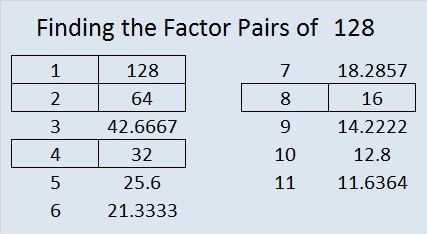128 is never a clue in the FIND THE FACTORS  puzzles.Excel file of puzzles and last week’s solutions: 10 Factors 2014-05-26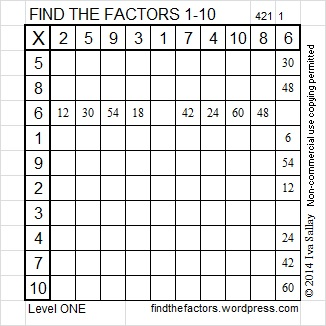# 126 and Level 5

126 is a composite number. 126 = 1 x 126, 2 x 63, 3 x 42, 6 x 21, 7 x 18, or 9 x 14. Factors of 126: 1, 2, 3, 6, 7, 9, 14, 18, 21, 42, 63, 126. Prime factorization: 126 = 2 x 3 x 3 x 7, which can also be written 2 x 3² x 7.Thinking process using divisibility tricks to find the factor pairs of 126 quickly:

√126 is irrational and approximately equal to 11.22. Every factor pair of 126 will have one factor less than 11.22 and one factor greater than 11.22, and we will find both factors in each pair at the same time. The following integers are less than 11.22. Are they factors of 126?

1. Yes, all whole numbers are divisible by 1, so 1 x 126 = 126.
2. Yes, 126 is an even number. 126 ÷ 2 = 63, so 2 x 63 = 126. (Since 63 is odd, 4 will not be a factor of 126.)
3. Yes, 1 + 2 + 6 = 9 which is divisible by both 3 and 9, so 126 is divisible by both 3 and 9, and 3 x 42 = 126.
4. No, the number formed from its last two digits, 26, isn’t divisible by 4, so 126 is not divisible by 4.
5. No, the last digit is not 0 or 5, so 126 is not divisible by 5.
6. Yes, 126 is divisible by both 2 and 3, so it is divisible by 6, and 6 x 21 = 126.
7. Yes, 63, 42, and 21 found above give this fact away. But also try the divisibility trick for 7 which requires us to split 126 into 12 and 6. We double 6 and subtract the double from 12. We get 12 – (2 x 6) = 12 – 12 = 0. Since 0 is divisible by 7, 126 is also divisible by 7, and 7 x 18 = 126.
8. No, since 126 isn’t divisible by 4, it is not divisible by any multiple of 4.
9. Yes, see 3 above. 126 is divisible by 9, and 9 x 14 = 126.
10. No, 126 doesn’t ends with a zero, so 10 is not a factor of 126.
11. No. I’ve typed the digits that are in an odd position in regular type and the digit in an even position in bold type: 126. To perform the divisibility trick for 11, we find the sum of the numbers typed in regular type (1 + 6  = 7) and the sum of the numbers typed in bold type (2) and subtract the smaller sum from the larger sum (7 – 2 = 5). If the difference were a multiple of 11, than the original number would also be a  multiple of 11 (and divisible by 11). Since 5 is not a multiple of 11, we know 126 is not divisible by 11.

From this thinking process we conclude that the factor pairs of 126 are 1 x 126, 2 x 63, 3 x 42, 6 x 21, 7 x 18, and 9 x 14.

126 is never a clue in the FIND THE FACTORS puzzles.

Today’s puzzle might be the most difficult level 5 puzzle I have ever published, so if you have never solved a level 5 or level 6 puzzle before, you probably don’t want to start with this one.Excel file of this week’s puzzles and last week’s factors: 12 Factors 2014-05-19